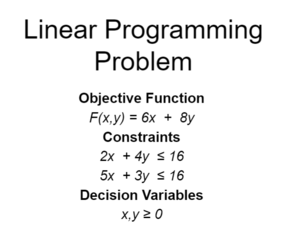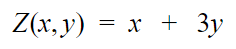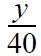# Linear Programming ProblemLinear Programming

Linear Programming is a tool that can be used to deal with problems in a large number of areas including military applications, transportation and distribution, scheduling, production and inventory management, telecommunication, agriculture and household management of income and expenditure.

Linear Programming was developed in World War II and the term “programming” referred to activities such as planning schedules or deploying men efficiently and optimally. It defines the process of selecting the best solution from various alternatives.

In Mathematics, Linear Programming is defined as a procedure of determining the most favourable outcome (or maximum or minimum distributable value) of a certain function (i.e. objective function) under certain given conditions (i.e. constraints).

Linear Programming Problem

Linear Programming Problems are problems of maximizing or minimizing a linear function of variables subjected to the conditions imposed on it.

Terms

Objective Function: The expression being optimized in a linear programming problem is called the objective function. The linear function of which we are required to find the maximum or minimum value is the objective function itself.

Constraints: The conditions imposed on the linear function (objective function) which are to be satisfied are the constraints.

Decision Variables: The non-negative independent variables used to give the ultimate solution of linear programming problems are decision variables. They may be material, men, machines, etc.

Optimal Value: The desired largest (or smallest) value of the objective function is called the optimal value.

Optimal Solution: The collection of values of the variables that give the optimal value is known as the optimal solution.

Thus, Linear Programming Problems are problems concerned with finding the optimal value of the given objective function subjected to its constraints.

Mathematical Formulation of the Problem

1. Identify decision variables and assign symbols.

2. Examine constraints to be satisfied and express in terms of equations or inequalities.

3. Decide the objective function and express it in the form of a linear function.

Mathematical Model of Linear Programming Problemsubject to constraints,The linear function Z = ax + by which is to be optimized is the objective function.

The conditions imposed (i) are the constraints.

The variables x and y are the decision variables

The conditions x,y ≥ 0 are the non-negativity constraints of the decision variables.

A typical maximization problem in two variables:

Maximize:subject to constraints,A typical minimization problem in two variables:

Minimize:subject to constraints,Exercise:

(Formulating a LPP)

If a man rides his car at 25 km/hr, he has to spend Rs. 2 per km on petrol, if he rides it at a faster speed of 40 km/hr, the petrol cost increases to Rs. 5 per km. He has Rs. 100 to spend on petrol and wishes to find the maximum distance he can travel within 1 hour. Formulate the above problem.

Solution:

Let the distance covered in two different cases be x km and y km.

Objective function F(x,y) = x + y

Now,

Cost of petrol per x km at Rs. 2 per km = 2x

Cost of petrol per y km at Rs. 5 per km = 5y

The man has Rs. 100 to spend for petrol

∴ 2x + 5y ≤ 100

And,

Time to travel x km at 25 km/ hr =Time to travel y km at 40 km/ hr =Total available time = 1 hr∴ 8x + 5y ≤ 200

Since, distance can never be negative,

∴ x,y ≥ 0

Total distance he can travel is, (x+y) km.

Hence, the linear programming model is,

Max. Z= x+y

subject to constraints,

2x + 5y ≤ 100

8x + 5y ≤ 200

x,y ≥ 0

﻿Bottom Line:

﻿﻿Solving a LP problem can be done in various ways. One easy way is the graphical method and the other is simplex method.